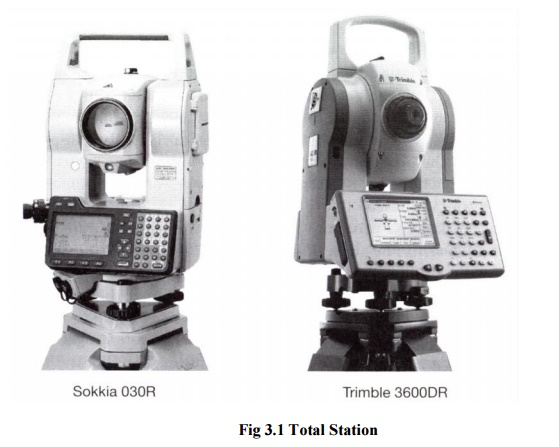Home | | Surveying II | | Surveying | Total Station: Basic Principle

# Total Station: Basic PrincipleAlthough taping and theodolites are used regularly on site - total stations are also used extensively in surveying, civil engineering and construction because they can measure both distances and angles.

TOTAL STATION: BASIC PRINCIPLE

Although taping and theodolites are used regularly on site - total stations are also used extensively in surveying, civil engineering and construction because they can measure both distances and angles.

A typical total station is shown in the figure belowFig 3.1 Total Station

Because the instrument combines both angle and distance measurement in the same unit, it is known as an integrated total station which can measure horizontal and vertical angles as well as slope distances.

Using the vertical angle, the total station can calculate the horizontal and vertical distance components of the measured slope distance.

As well as basic functions, total stations are able to perform a number of different survey tasks and associated calculations and can store large amounts of data.

As with the electronic theodolite, all the functions of a total station are controlled by its microprocessor, which is accessed thought a keyboard and display.

To use the total station, it is set over one end of the line to be measured and some reflector is positioned at the other end such that the line of sight between the instrument and the reflector is unobstructed (as seen in the figure below).

-The reflector is a prism attached to a detail pole

-The telescope is aligned and pointed at the prism

-The measuring sequence is initiated and a signal is sent to the reflector and a part of this signal is returned to the total station

-This signal is then analysed to calculate the slope distance together with the horizontal and vertical angles.

-Total stations can also be used without reflectors and the telescope is pointed at the point that needs to be measured

-Some instruments have motorised drivers and can be use automatic target recognition to search and lock into a prism - this is a fully automated process and does not require an operator.

-Some total stations can be controlled from the detail pole, enabling surveys to be conducted by one person.Fig 3.2 Measuring with a Total Station

Most total stations have a distance measuring range of up to a few kilometres, when using a prism, and a range of at least 100m in reflector less mode and an accuracy of 2-3mm at short ranges, which will decrease to about 4-5mm at 1km.

Although angles and distances can be measured and used separately, the most common applications for total stations occur when these are combined to define position in control surveys.

As well as the total station, site surveying is increasingly being carried out using GPS equipment. Some predictions have been made that this trend will continue, and in the long run GPS methods may replace other methods.

Although the use of GPS is increasing, total stations are one of the predominant instruments used on site for surveying and will be for some time.

Developments in both technologies will find a point where devices can be made that complement both methods.

Study Material, Lecturing Notes, Assignment, Reference, Wiki description explanation, brief detail

Related Topics